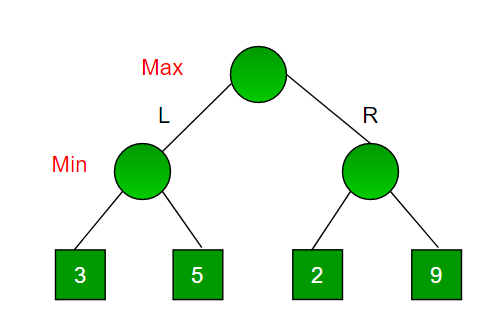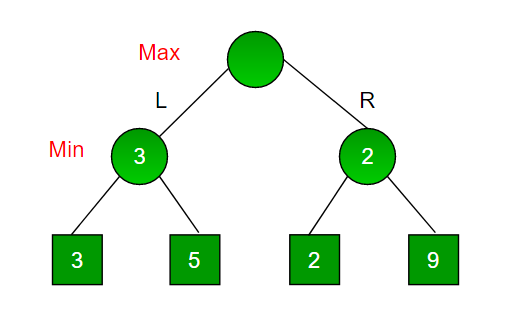GFG App
Open AppBrowser
Continue

# Minimax Algorithm in Game Theory | Set 1 (Introduction)

Minimax is a kind of backtracking algorithm that is used in decision making and game theory to find the optimal move for a player, assuming that your opponent also plays optimally. It is widely used in two player turn-based games such as Tic-Tac-Toe, Backgammon, Mancala, Chess, etc.
In Minimax the two players are called maximizer and minimizer. The maximizer tries to get the highest score possible while the minimizer tries to do the opposite and get the lowest score possible.
Every board state has a value associated with it. In a given state if the maximizer has upper hand then, the score of the board will tend to be some positive value. If the minimizer has the upper hand in that board state then it will tend to be some negative value. The values of the board are calculated by some heuristics which are unique for every type of game.

Example:
Consider a game which has 4 final states and paths to reach final state are from root to 4 leaves of a perfect binary tree as shown below. Assume you are the maximizing player and you get the first chance to move, i.e., you are at the root and your opponent at next level. Which move you would make as a maximizing player considering that your opponent also plays optimally?Since this is a backtracking based algorithm, it tries all possible moves, then backtracks and makes a decision.

• Maximizer goes LEFT: It is now the minimizers turn. The minimizer now has a choice between 3 and 5. Being the minimizer it will definitely choose the least among both, that is 3
• Maximizer goes RIGHT: It is now the minimizers turn. The minimizer now has a choice between 2 and 9. He will choose 2 as it is the least among the two values.

Being the maximizer you would choose the larger value that is 3. Hence the optimal move for the maximizer is to go LEFT and the optimal value is 3.

Now the game tree looks like below :The above tree shows two possible scores when maximizer makes left and right moves.

Note: Even though there is a value of 9 on the right subtree, the minimizer will never pick that. We must always assume that our opponent plays optimally.

Below is the implementation for the same.

## C++

 `// A simple C++ program to find` `// maximum score that` `// maximizing player can get.` `#include` `using` `namespace` `std;`   `// Returns the optimal value a maximizer can obtain.` `// depth is current depth in game tree.` `// nodeIndex is index of current node in scores[].` `// isMax is true if current move is` `// of maximizer, else false` `// scores[] stores leaves of Game tree.` `// h is maximum height of Game tree` `int` `minimax(``int` `depth, ``int` `nodeIndex, ``bool` `isMax,` `            ``int` `scores[], ``int` `h)` `{` `    ``// Terminating condition. i.e` `    ``// leaf node is reached` `    ``if` `(depth == h)` `        ``return` `scores[nodeIndex];`   `    ``//  If current move is maximizer,` `    ``// find the maximum attainable` `    ``// value` `    ``if` `(isMax)` `       ``return` `max(minimax(depth+1, nodeIndex*2, ``false``, scores, h),` `            ``minimax(depth+1, nodeIndex*2 + 1, ``false``, scores, h));`   `    ``// Else (If current move is Minimizer), find the minimum` `    ``// attainable value` `    ``else` `        ``return` `min(minimax(depth+1, nodeIndex*2, ``true``, scores, h),` `            ``minimax(depth+1, nodeIndex*2 + 1, ``true``, scores, h));` `}`   `// A utility function to find Log n in base 2` `int` `log2(``int` `n)` `{` `  ``return` `(n==1)? 0 : 1 + log2(n/2);` `}`   `// Driver code` `int` `main()` `{` `    ``// The number of elements in scores must be` `    ``// a power of 2.` `    ``int` `scores[] = {3, 5, 2, 9, 12, 5, 23, 23};` `    ``int` `n = ``sizeof``(scores)/``sizeof``(scores);` `    ``int` `h = log2(n);` `    ``int` `res = minimax(0, 0, ``true``, scores, h);` `    ``cout << ``"The optimal value is : "` `<< res << endl;` `    ``return` `0;` `}`

## Java

 `// A simple java program to find maximum score that` `// maximizing player can get.`   `import` `java.io.*;`   `class` `GFG {` `  `    `// Returns the optimal value a maximizer can obtain.` `// depth is current depth in game tree.` `// nodeIndex is index of current node in scores[].` `// isMax is true if current move is of maximizer, else false` `// scores[] stores leaves of Game tree.` `// h is maximum height of Game tree` ` ``static` `int` `minimax(``int` `depth, ``int` `nodeIndex, ``boolean`  `isMax,` `            ``int` `scores[], ``int` `h)` `{` `    ``// Terminating condition. i.e leaf node is reached` `    ``if` `(depth == h)` `        ``return` `scores[nodeIndex];`   `    ``// If current move is maximizer, find the maximum attainable` `    ``// value` `    ``if` `(isMax)` `    ``return` `Math.max(minimax(depth+``1``, nodeIndex*``2``, ``false``, scores, h),` `            ``minimax(depth+``1``, nodeIndex*``2` `+ ``1``, ``false``, scores, h));`   `    ``// Else (If current move is Minimizer), find the minimum` `    ``// attainable value` `    ``else` `        ``return` `Math.min(minimax(depth+``1``, nodeIndex*``2``, ``true``, scores, h),` `            ``minimax(depth+``1``, nodeIndex*``2` `+ ``1``, ``true``, scores, h));` `}`   `// A utility function to find Log n in base 2` ` ``static` `int` `log2(``int` `n)` `{` `return` `(n==``1``)? ``0` `: ``1` `+ log2(n/``2``);` `}`   `// Driver code`   `    ``public` `static` `void` `main (String[] args) {` `            ``// The number of elements in scores must be` `    ``// a power of 2.` `    ``int` `scores[] = {``3``, ``5``, ``2``, ``9``, ``12``, ``5``, ``23``, ``23``};` `    ``int` `n = scores.length;` `    ``int` `h = log2(n);` `    ``int` `res = minimax(``0``, ``0``, ``true``, scores, h);` `    ``System.out.println( ``"The optimal value is : "`  `+res); ` `        `  `    ``}` `}`   `// This code is contributed by vt_m`

## C#

 `// A simple C# program to find maximum score that` `// maximizing player can get.` `using` `System;`   `public` `class` `GFG` `{` `    `  `// Returns the optimal value a maximizer can obtain.` `// depth is current depth in game tree.` `// nodeIndex is index of current node in scores[].` `// isMax is true if current move is of maximizer, else false` `// scores[] stores leaves of Game tree.` `// h is maximum height of Game tree` `static` `int` `minimax(``int` `depth, ``int` `nodeIndex, ``bool` `isMax,` `            ``int` `[]scores, ``int` `h)` `{` `    ``// Terminating condition. i.e leaf node is reached` `    ``if` `(depth == h)` `        ``return` `scores[nodeIndex];`   `    ``// If current move is maximizer, find the maximum attainable` `    ``// value` `    ``if` `(isMax)` `    ``return` `Math.Max(minimax(depth+1, nodeIndex*2, ``false``, scores, h),` `            ``minimax(depth+1, nodeIndex*2 + 1, ``false``, scores, h));`   `    ``// Else (If current move is Minimizer), find the minimum` `    ``// attainable value` `    ``else` `        ``return` `Math.Min(minimax(depth+1, nodeIndex*2, ``true``, scores, h),` `            ``minimax(depth+1, nodeIndex*2 + 1, ``true``, scores, h));` `}`   `// A utility function to find Log n in base 2` `static` `int` `log2(``int` `n)` `{` `    ``return` `(n==1)? 0 : 1 + log2(n/2);` `}`   `// Driver code` `static` `public` `void` `Main ()` `{`   `    ``// The number of elements in scores must be` `    ``// a power of 2.` `    ``int` `[]scores = {3, 5, 2, 9, 12, 5, 23, 23};` `    ``int` `n = scores.Length;` `    ``int` `h = log2(n);` `    ``int` `res = minimax(0, 0, ``true``, scores, h);` `    ``Console.WriteLine( ``"The optimal value is : "` `+res); ` `    `  `}` `}`   `// This code is contributed by ajit.`

## Python3

 `# A simple Python3 program to find` `# maximum score that` `# maximizing player can get` `import` `math`   `def` `minimax (curDepth, nodeIndex,` `             ``maxTurn, scores, ` `             ``targetDepth):`   `    ``# base case : targetDepth reached` `    ``if` `(curDepth ``=``=` `targetDepth): ` `        ``return` `scores[nodeIndex]` `    `  `    ``if` `(maxTurn):` `        ``return` `max``(minimax(curDepth ``+` `1``, nodeIndex ``*` `2``, ` `                    ``False``, scores, targetDepth), ` `                   ``minimax(curDepth ``+` `1``, nodeIndex ``*` `2` `+` `1``, ` `                    ``False``, scores, targetDepth))` `    `  `    ``else``:` `        ``return` `min``(minimax(curDepth ``+` `1``, nodeIndex ``*` `2``, ` `                     ``True``, scores, targetDepth), ` `                   ``minimax(curDepth ``+` `1``, nodeIndex ``*` `2` `+` `1``, ` `                     ``True``, scores, targetDepth))` `    `  `# Driver code` `scores ``=` `[``3``, ``5``, ``2``, ``9``, ``12``, ``5``, ``23``, ``23``]`   `treeDepth ``=` `math.log(``len``(scores), ``2``)`   `print``(``"The optimal value is : "``, end ``=` `"")` `print``(minimax(``0``, ``0``, ``True``, scores, treeDepth))`   `# This code is contributed` `# by rootshadow`

## Javascript

 ``

Output:

`The optimal value is:  12`

Time complexity : O(b^d) b is the branching factor and d is count of depth or ply of graph or tree.

Space Complexity : O(bd) where b is branching factor into d is maximum depth of tree similar to DFS.